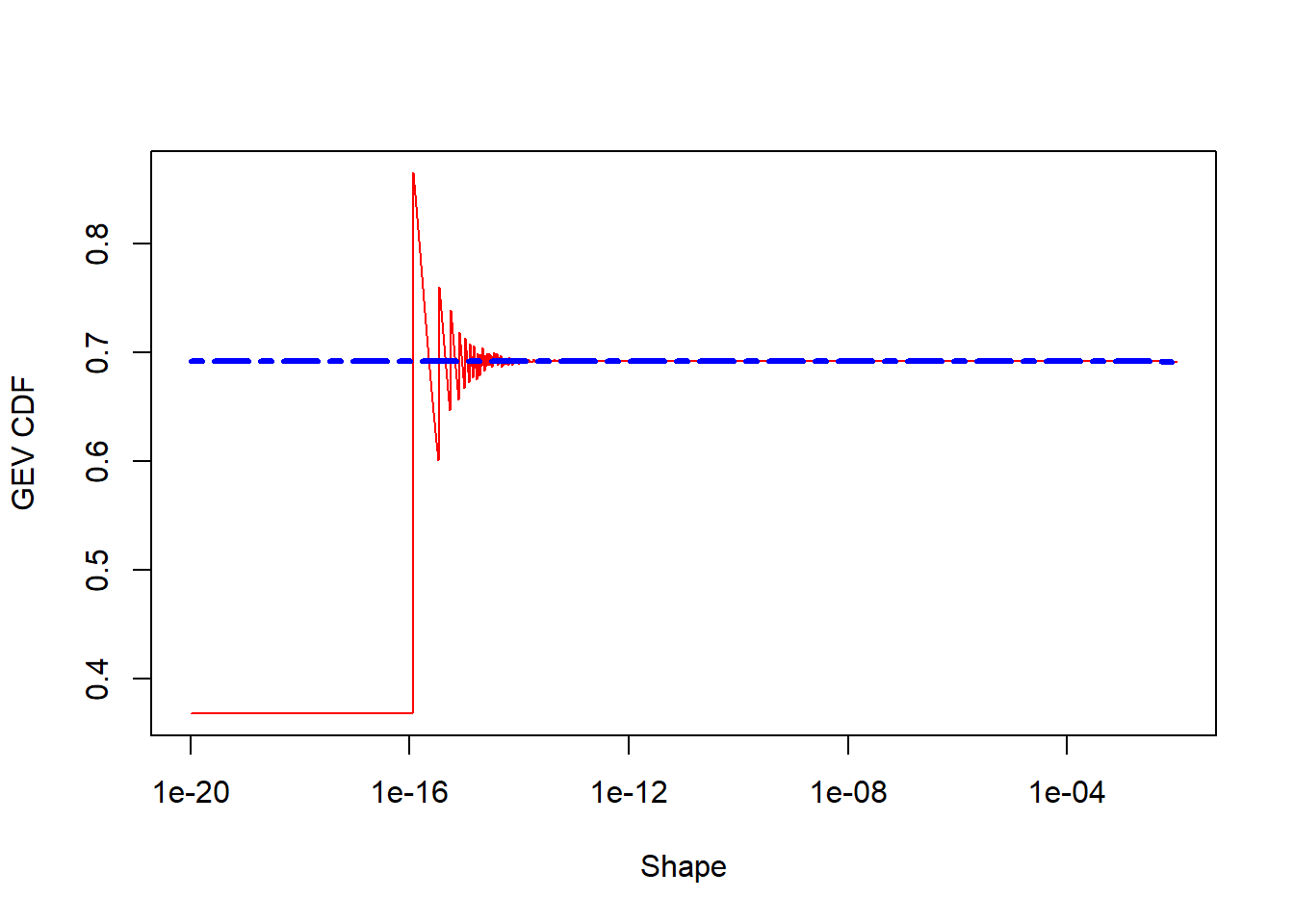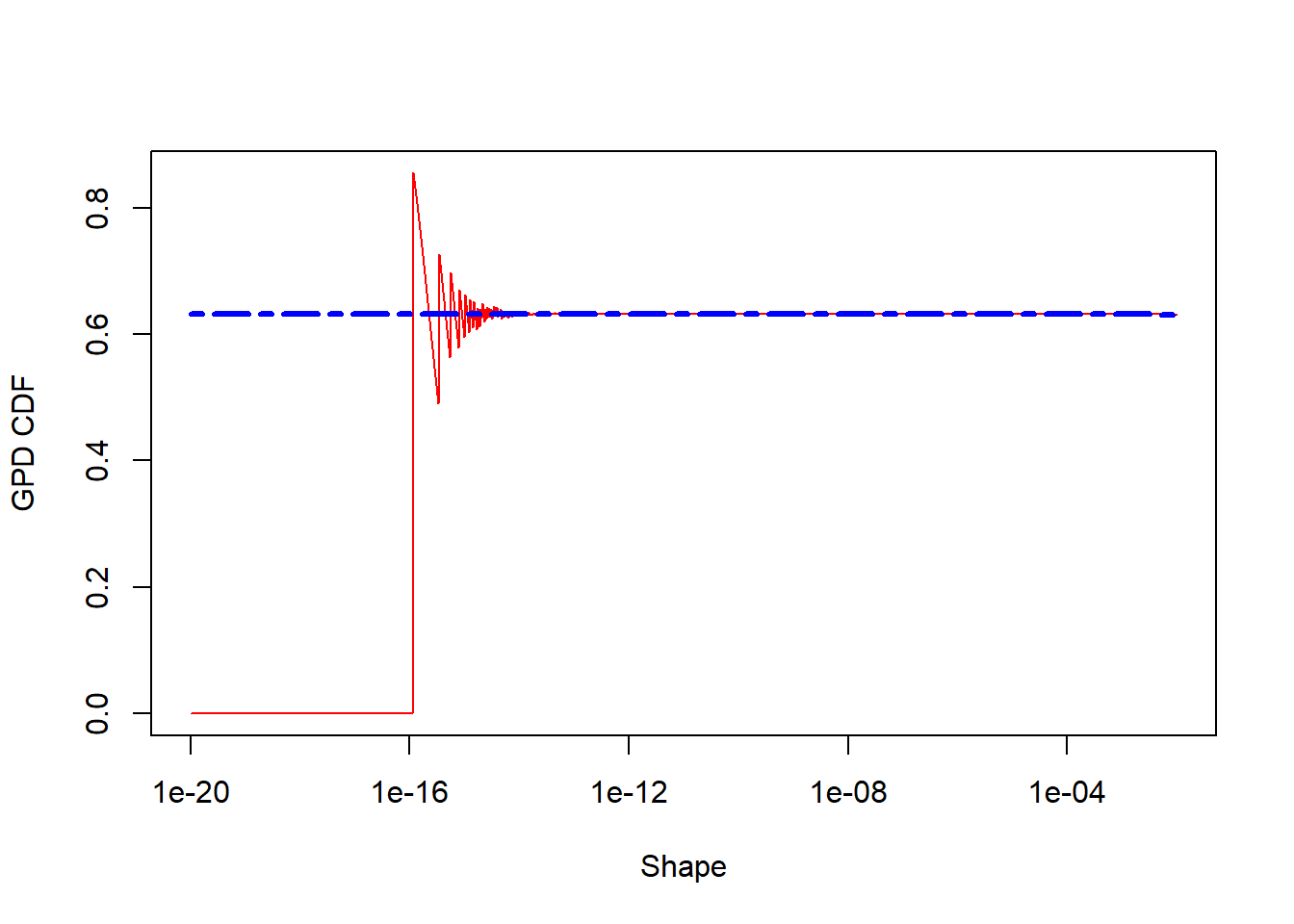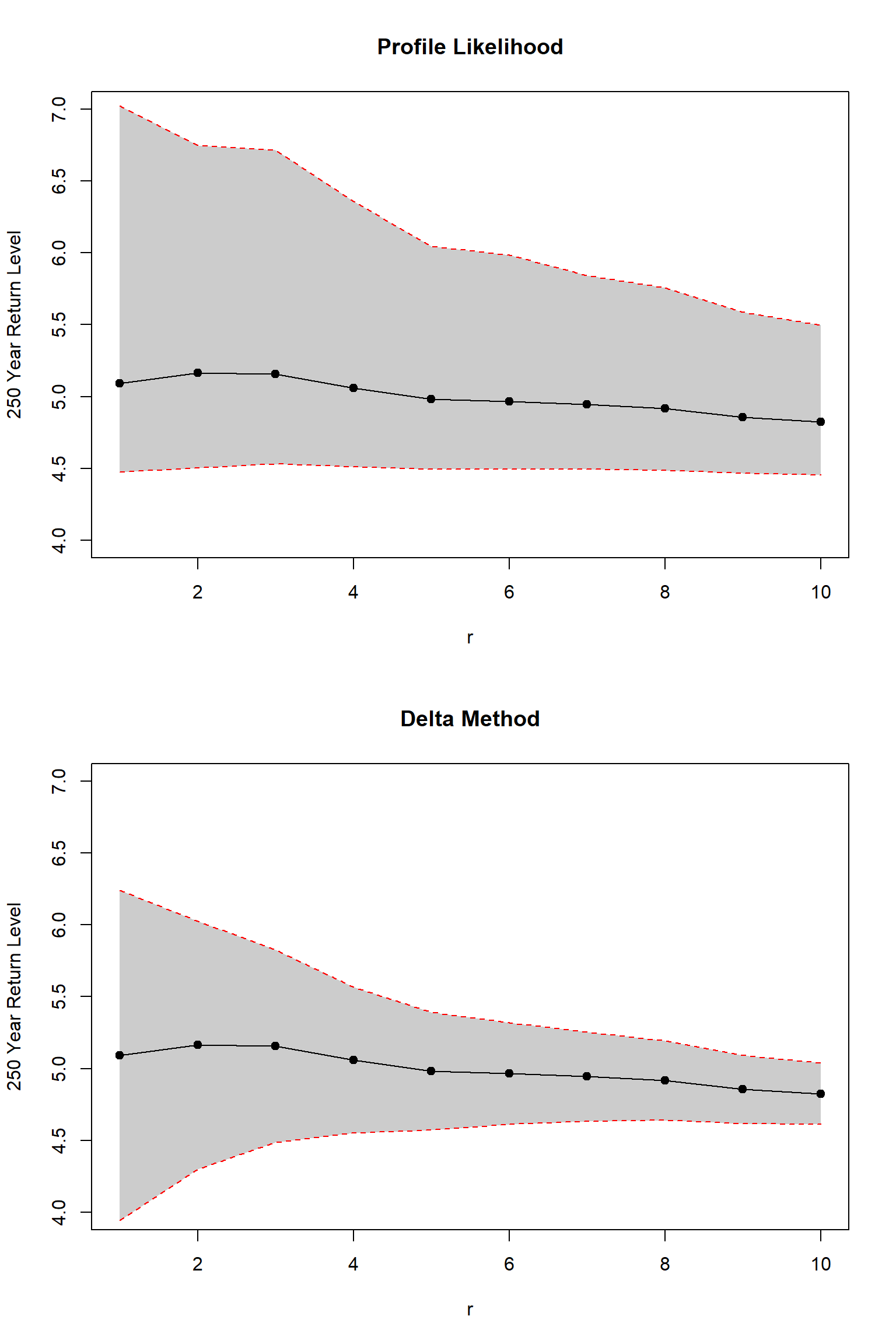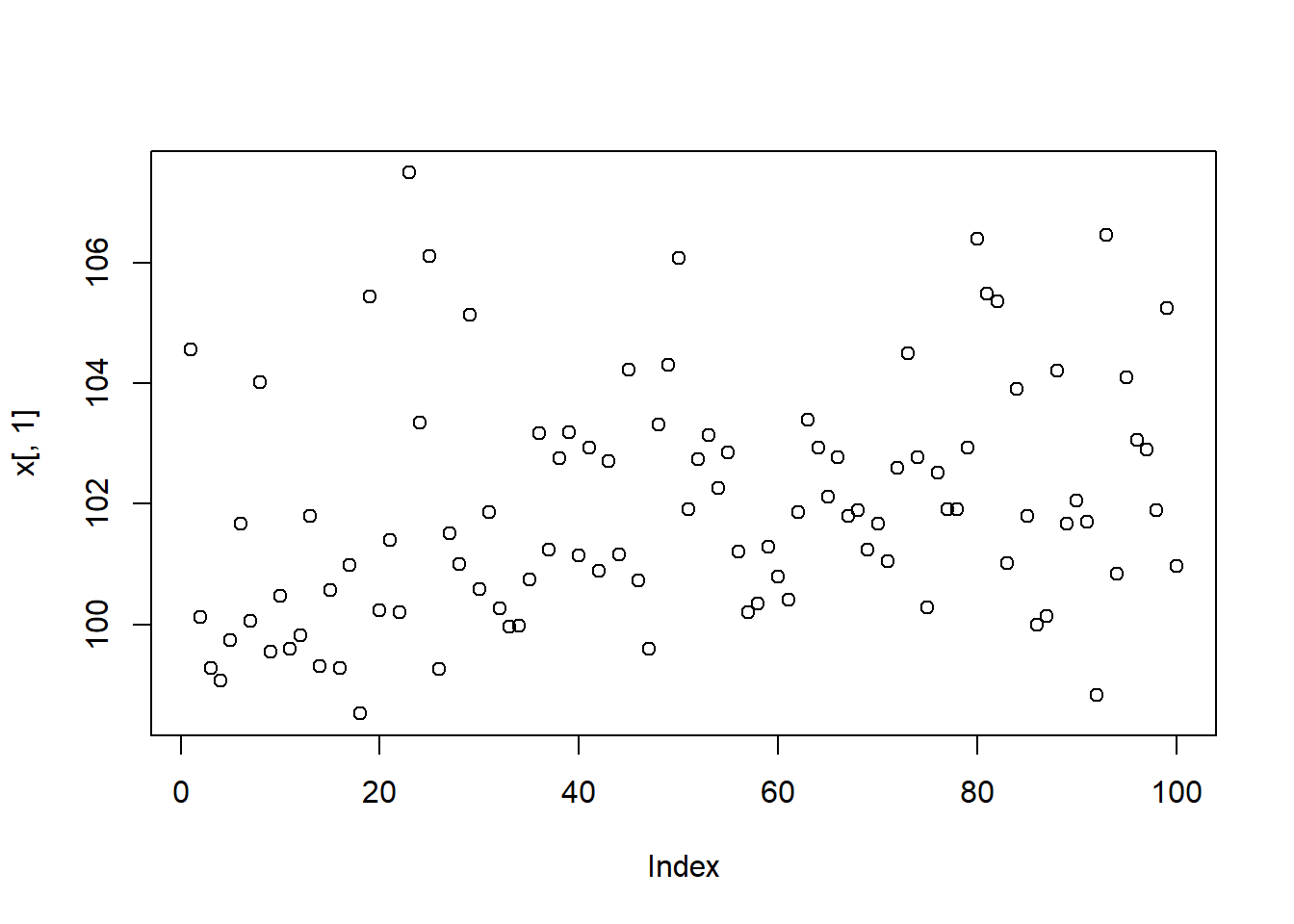title: “Introduction to ‘eva’ and its capabilities” author: Brian Bader date: “2020-11-14” output: rmarkdown::html_vignette vignette: > % %

The eva Package

The ‘eva’ package, short for ‘Extreme Value Analysis with Goodness-of-Fit Testing’, provides functionality that allows data analysis of extremes from beginning to end, with model fitting and a slew of newly available tests for diagnostics. In particular, some highlights are:

• Implementation of the $$r$$ largest order statistics (GEV$$_r$$) model - data generation, fitting, and return levels.

• Efficient handling of the near-zero shape parameter.

• Maximum product spacings (MPS) estimation for parameters in the block maxima (GEV$$_1$$) and generalized pareto distributions.

• Sequential tests for the choice of $$r$$ in the GEV$$_r$$ model, as well as tests for the selection of threshold in the peaks-over-threshold (POT) approach. For the boostrap based tests, the option to run in parallel is provided.

• P-value adjustments to control for the false discover rate (FDR) and family-wise error rate (FWER) in the sequential testing setting.

Efficient handling of near-zero shape parameter

library(eva)

# A naive implementation of the GEV cumulative density function
pgev_naive <- function(q, loc = 0, scale = 1, shape = 1) {
exp(-(1 + (shape * (q - loc))/scale)^(-1/shape))
}

curve(pgev_naive(1, 0, 1, x), 1e-20, .01, log = "x", n = 1025,
xlab = "Shape", ylab = "GEV CDF", col = "red", lty = 1, lwd = 1)
curve(pgev(1, 0, 1, x), 1e-20, .01, log = "x", n = 1025, col = "blue", lty = 6, lwd = 3, add = TRUE)# Similarly for the GPD cdf
pgpd_naive <- function(q, loc = 0, scale = 1, shape = 1) {
(1 - (1 + (shape * (q - loc))/scale)^(-1/shape))
}

curve(pgpd_naive(1, 0, 1, x), 1e-20, .01, log = "x", n = 1025,
xlab = "Shape", ylab = "GPD CDF", col = "red", lty = 1, lwd = 1)
curve(pgpd(1, 0, 1, x),  1e-20, .01, log = "x", n = 1025, col = "blue", lty = 6, lwd = 3, add = TRUE)The GEV$$_r$$ distribution

The GEV$$_r$$ distribution has the density function $f_r (x_1, x_2, ..., x_r | \mu, \sigma, \xi) = \sigma^{-r}\exp\left\{-(1+\xi z_r)^{-\frac{1}{\xi}} - \left(\frac{1}{\xi}+1\right)\sum_{j=1}^{r}\log(1+\xi z_j)\right\}$ for some location parameter $$\mu$$, scale parameter $$\sigma > 0$$ and shape parameter $$\xi$$, where $$x_1 > \cdots> x_r$$, $$z_j = (x_j - \mu) / \sigma$$, and $$1 + \xi z_j > 0$$ for $$j=1, \ldots, r$$. When $$r = 1$$, this distribution is exactly the GEV distribution or block maxima.

This package includes data generation (rgevr), density function (dgevr), fitting (gevrFit), and return levels (gevrRl) for this distribution. If one wants to choose $$r > 1$$, goodness-of-fit must be tested. This can be done using function gevrSeqTests. Take, for example, the dataset Lowestoft, which includes the top ten sea levels at Lowestoft harbor from 1964 - 2014. Two tests are available to run in sequence - the entropy difference and score test.

data(lowestoft)
gevrSeqTests(lowestoft, method = "ed")
##    r  p.values ForwardStop StrongStop   statistic  est.loc est.scale  est.shape
## 1  2 0.9774687    1.455825  0.9974711  0.02824258 3.431792 0.2346591 0.10049739
## 2  3 0.7747741    1.163697  1.0869254 -0.28613586 3.434097 0.2397408 0.09172687
## 3  4 0.5830318    1.116989  1.1500564  0.54896156 3.447928 0.2404563 0.06802070
## 4  5 0.6445475    1.157363  1.2470248  0.46135009 3.452449 0.2376723 0.05451138
## 5  6 0.4361569    1.181963  1.2676077 -0.77869930 3.455478 0.2396332 0.04709329
## 6  7 0.6329943    1.334209  1.4133341  0.47751655 3.454680 0.2372572 0.04555449
## 7  8 0.4835074    1.444819  1.4790559 -0.70067260 3.455901 0.2376215 0.03838020
## 8  9 0.8390270    1.836882  2.0321878 -0.20313820 3.458135 0.2356543 0.02536685
## 9 10 0.8423291    1.847245  3.4235418  0.19891516 3.459470 0.2342272 0.01964612

The entropy difference test fails to reject for any value of $$r$$ from 1 to 10. A common quantity of interest in extreme value analysis are the $$m$$-year return levels, which can be thought of as the average maximum value that will be exceeded over a period of $$m$$ years. For the Lowestoft data, the 250 year sea level return levels, with 95% confidence intervals are plotted for $$r$$ from 1 to 10. The advantage of using more top order statistics can be seen in the plots below. The width of the intervals decrease by over two-thirds from $$r=1$$ to $$r=10$$. Similarly decreases can be seen in the parameter intervals.

# Make 250 year return level plot using gevr for r = 1 to 10 with the LoweStoft data

data(lowestoft)
result <- matrix(0, 20, 4)
period <- 250

for(i in 1:10) {
z <- gevrFit(as.matrix(lowestoft[, 1:i]))
y1 <- gevrRl(z, period, conf = 0.95, method = "delta")
y2 <- gevrRl(z, period, conf = 0.95, method = "profile", plot = FALSE)
result[i, 1] <- i
result[i, 2] <- y1$Estimate result[i, 3:4] <- y1$CI
result[(i + 10), 1] <- i
result[(i + 10), 2] <- y2$Estimate result[(i + 10), 3:4] <- y2$CI
}

result <- cbind.data.frame(result, c(rep("Delta", 10), rep("Profile", 10)))
colnames(result) <- c("r", "Est", "Lower", "Upper", "Method")
result <- as.data.frame(result)

prof <- subset(result, Method == "Profile")
del <- subset(result, Method == "Delta")

par(mfrow = c(2, 1))

plot(prof$r, prof$Est, main = "Profile Likelihood",
xlab = "r", ylab = "250 Year Return Level",
xlim = c(1, 10), ylim = c(4, 7))
polygon(c(rev(prof$r), prof$r), c(rev(prof$Lower), prof$Upper), col = 'grey80', border = NA)
points(prof$r, prof$Est, pch = 19, col = 'black')
lines(prof$r, prof$Est, lty = 'solid', col = 'black')
lines(prof$r, prof$Lower, lty = 'dashed', col = 'red')
lines(prof$r, prof$Upper, lty = 'dashed', col = 'red')

plot(del$r, del$Est, main = "Delta Method",
xlab = "r", ylab = "250 Year Return Level",
xlim = c(1, 10), ylim = c(4, 7))
polygon(c(rev(del$r), del$r), c(rev(del$Lower), del$Upper), col = 'grey80', border = NA)
points(del$r, del$Est, pch = 19, col = 'black')
lines(del$r, del$Est, lty = 'solid', col = 'black')
lines(del$r, del$Lower, lty = 'dashed', col = 'red')
lines(del$r, del$Upper, lty = 'dashed', col = 'red')par(oldpar)

In addition, the profile likelihood confidence intervals are compared with the delta method intervals. The advantage of using profile likelihood over the delta method is the allowance for asymmetric intervals. This is especially useful at high quantiles, or large return level periods. In the Lowestoft plots directly above, the asymmetry can be seen in the stable lower bound across values of $$r$$, while the upper bound decreases.

Fitting the GEV$$_r$$ distribution

set.seed(7)
n <- 100
r <- 10
x <- rgevr(n, r, loc = 100 + 1:n / 50,  scale = 1 + 1:n / 100, shape = 0)

## Plot the largest order statistic
plot(x[, 1])## Creating covariates (linear trend first)
covs <- as.data.frame(seq(1, n, 1))
names(covs) <- c("Trend1")
## Create some unrelated covariates
covs$Trend2 <- rnorm(n) covs$Trend3 <- 30 * runif(n)

## Use full data
fit_full <- gevrFit(data = x, method = "mle", locvars = covs, locform = ~ Trend1 + Trend2*Trend3,
scalevars = covs, scaleform = ~ Trend1)

## Only use r = 1
fit_top_only <- gevrFit(data = x[, 1], method = "mle", locvars = covs, locform = ~ Trend1 + Trend2*Trend3,
scalevars = covs, scaleform = ~ Trend1)

In the previous chunk of code, we look at non-stationary fitting in the GEV$$_r$$ distribution. The ‘eva’ package allows generalized linear modeling in each parameter (location, scale, and shape), as well as specifying specific link functions. As opposed to some other packages, one can use formulas when specifying the models, so it is quite user friendly. Additionally, to benefit optimization, there is efficient handling of the near-zero shape parameter in the likelihood and covariates are automatically centered and scaled when appropriate (they are transformed back to the original scale in the output).

The previous chunk of code demonstrates the benefit of using a larger $$r$$ than just the block maxima. Data is generated with sample size 100 and $$r=10$$, with a linear trend in both the location and scale parameter. From a visual assessment of the largest order statistic, it is difficult to see either trend in the data. We include two erroneous covariates, named ‘Trend2’ and ‘Trend3’, and fit the nonstationary distribution with the full data ($$r=10$$) and only the block maxima ($$r=1$$). The results are summarized in the gevrFit function.

## Show summary of estimates
fit_full
## Summary of fit:
##                           Estimate Std. Error   z value   Pr(>|z|)
## Location (Intercept)   100.0856115  0.2276723 439.60378 0.0000e+00 ***
## Location Trend1          0.0193838  0.0042366   4.57535 4.7543e-06 ***
## Location Trend2         -0.0716415  0.0949454  -0.75455 4.5052e-01
## Location Trend3          0.0031478  0.0049866   0.63124 5.2788e-01
## Location Trend2:Trend3   0.0032205  0.0053048   0.60710 5.4378e-01
## Scale (Intercept)        1.0831201  0.0922684  11.73879 8.0627e-32 ***
## Scale Trend1             0.0097441  0.0016675   5.84352 5.1108e-09 ***
## Shape (Intercept)        0.0393603  0.0293665   1.34031 1.8014e-01
## ---
## Signif. codes:  0 '***' 0.001 '*' 0.01 '*' 0.05 '.' 0.1 ' ' 1
fit_top_only
## Summary of fit:
##                          Estimate Std. Error   z value   Pr(>|z|)
## Location (Intercept)   99.5896128  0.4373915 227.68985 0.0000e+00 ***
## Location Trend1         0.0235401  0.0052891   4.45072 8.5582e-06 ***
## Location Trend2        -0.3567545  0.2858703  -1.24796 2.1205e-01
## Location Trend3         0.0170264  0.0170313   0.99971 3.1745e-01
## Location Trend2:Trend3  0.0021764  0.0194109   0.11212 9.1073e-01
## Scale (Intercept)       1.1098238  0.2654124   4.18151 2.8958e-05 ***
## Scale Trend1            0.0036369  0.0042099   0.86390 3.8764e-01
## Shape (Intercept)       0.1375594  0.1063641   1.29329 1.9591e-01
## ---
## Signif. codes:  0 '***' 0.001 '*' 0.01 '*' 0.05 '.' 0.1 ' ' 1

From the output, one can see from the fit summary that using $$r=10$$, both the correct trend in location and scale are deemed significant. However, using $$r=1$$ a test for significance in the scale trend fails.

Next, we fit another two models (using $$r = 10$$). The first, labeled ‘fit_reduced1’ is a GEV$$_{10}$$ fit incorporating only the true linear trends as covariates in the location and scale parameters. The second, ‘fit_reduced2’ fits the Gumbel equivalent of this model ($$\xi = 0$$). Note that ‘fit_reduced1’ is nested within ‘fit_full’ and ‘fit_reduced2’ is further nested within ‘fit_reduced1’.

One way to compare these models is using the Akaike information criterion (AIC) and choose the model with the smallest value. This metric can be extracted by using AIC($$\cdot$$) on a gevrFit object. By this metric, the chosen model is ‘fit_reduced2’, which agrees with the true model (Gumbel, with linear trends in the location and scale parameters). We can also test between nested models using the likelihood ratio test (LRT). For example, the test of $$H_0$$: $$\xi = 0$$ between ‘fit_reduced1’ and ‘fit_reduced2’ is approximately distributed as chi-square with one degree of freedom and its p-value is found to be 0.95. Thus, the further reduced (Gumbel) model ‘fit_reduced2’ is favored.

## Compare AIC of three models
fit_reduced1 <- gevrFit(data = x, method = "mle", locvars = covs, locform = ~ Trend1,
scalevars = covs, scaleform = ~ Trend1)

fit_reduced2 <- gevrFit(data = x, method = "mle", locvars = covs, locform = ~ Trend1,
scalevars = covs, scaleform = ~ Trend1, gumbel = TRUE)

AIC(fit_full)
##  127.0764
AIC(fit_reduced1)
##  120.4856
AIC(fit_reduced2)
##  118.4895
LRT <- as.numeric(2 * (logLik(fit_reduced1) - logLik(fit_reduced2)))

pval <- pchisq(LRT, df = 1, ncp = 0, lower.tail = FALSE, log.p = FALSE)

round(pval, digits = 3)
##  0.95

One can also use the ‘gevrFit’ function to estimate the parameters of a multivariate model with dependence between GEV marginal distributions using independence likelihood. Here we generate correlation using the multivariate normal distribution and then transform the marginal distributions into GEV.

set.seed(7)
require(SpatialExtremes)
##
## Attaching package: 'SpatialExtremes'
## The following objects are masked from 'package:eva':
##
##     dgpd, pgev, pgpd, qgev, qgpd, rgpd
n.site <- 4
n.obs <- 15
## Simulate a max-stable random field
locations <- matrix(runif(2 * n.site, 0, 10), ncol = 2)
colnames(locations) <- c("lon", "lat")
## Smith model
U <- rmaxstab(n.obs, locations, "gauss", cov11 = 16, cov12 = 0, cov22 = 16)
cor(U, method = "spearman")
##           [,1]      [,2]      [,3]      [,4]
## [1,] 1.0000000 0.2857143 0.1250000 0.2714286
## [2,] 0.2857143 1.0000000 0.6964286 0.7714286
## [3,] 0.1250000 0.6964286 1.0000000 0.6500000
## [4,] 0.2714286 0.7714286 0.6500000 1.0000000

The previous chunk of code generates data from a multivariate max stable random variable on the Frechet scale. We can see the correlation is somewhat large. Next, we transform the uniform data into GEV margins with site specific location means [8, 10, 12, 9] and shared scale, trend parameters. The design matrix labels each of the four sites as 1, 2, 3, or 4. The estimated means can be constructed from the output. Site 1 is chosen as the reference level, so its location parameter is estimated by the location intercept – 8.12. The other site specific location parameter estimates can be obtained by adding the intercept and the corresponding site value. For example, site 3 has a location estimate of 8.12 + 4.02 = 12.14.

## Transform to GEV margins
locations <- c(8, 10, 12, 9)
out <- frech2gev(U, loc = 0, scale = 1, shape = 0.2)
out <- out + t(matrix(rep(locations, nrow(out)), ncol = nrow(out)))
out <- as.vector(out)

## Create design matrix for the location parameters
loc <- cbind.data.frame(as.factor(sort(rep(seq(1, n.site, 1), n.obs))))
colnames(loc) <- c("Site")

z <- gevrFit(out, locvars = loc, locform = ~ Site)
z
## Summary of fit:
##                       Estimate Std. Error   z value    Pr(>|z|)
## Location (Intercept) 8.1197181    0.32172 25.238373 1.5196e-140 ***
## Location Site2       1.9309253    0.37440  5.157381  2.5043e-07 ***
## Location Site3       4.0150971    0.39637 10.129764  4.0763e-24 ***
## Location Site4       0.9416902    0.39483  2.385056  1.7077e-02   *
## Scale (Intercept)    0.9465077    0.10922  8.666143  4.4700e-18 ***
## Shape (Intercept)    0.0095865    0.12631  0.075896  9.3950e-01
## ---
## Signif. codes:  0 '***' 0.001 '*' 0.01 '*' 0.05 '.' 0.1 ' ' 1